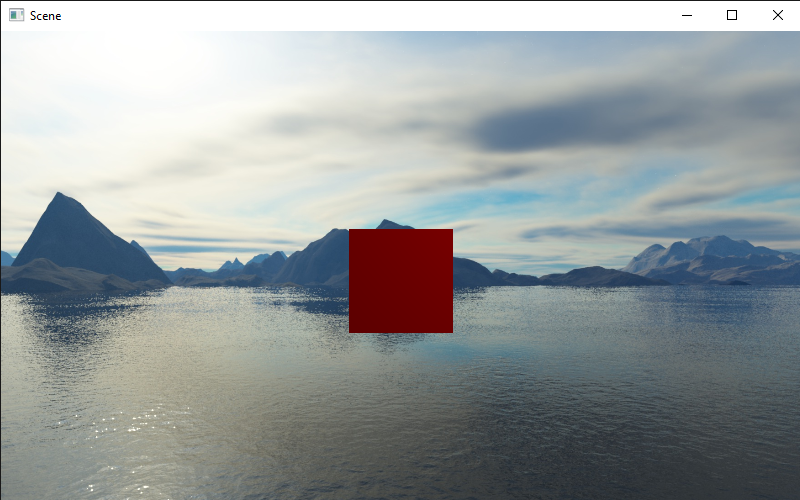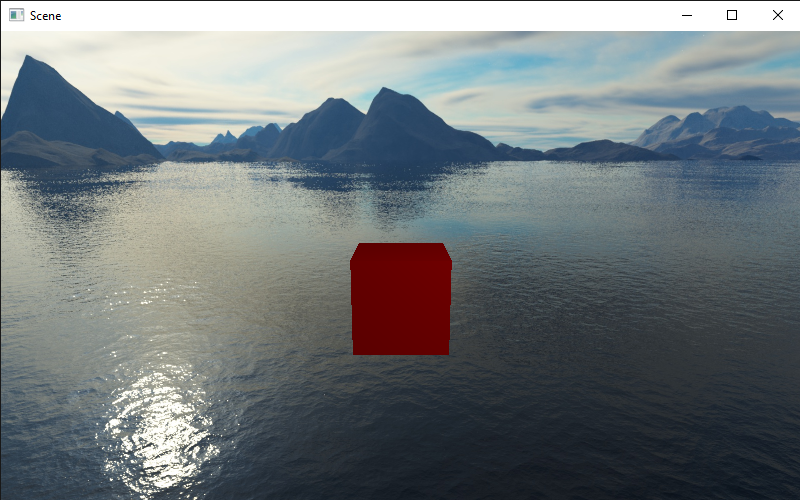# Tutorial 2: Rendering in Scenes¶

Last tutorial we covered some basic concepts on GameObjects and Transforms, and this time we’ll be looking at how to render things in a window.

## Scenes¶

A `Scene` is like a page to draw on: you can add things, remove things and change things. To create a scene, you can call `SceneManager.AddScene`:

```>>> scene = SceneManager.AddScene("Scene")
```

In your newly created scene, you have 2 GameObjects: a Main Camera, and a Light. These two things can be moved around like normal GameObjects.

Next, let’s move the camera back 10 units:

```>>> scene.mainCamera.transform.localPosition = Vector3(0, 0, -10)
```

`scene.mainCamera` references the `Camera` Component on the Main Camera, so we can access the Transform by using its `transform` attribute.

## Meshes¶

To render anything, we need a model of it. Let’s say we want to create a cube. Then we need a model of a cube, or what’s called a mesh. Meshes have 4 pieces of data: the vertices (or points), the faces, the normals and the texture coordinates. Normals are just vectors saying which way the face is pointing, and texture coordinates are coordinates to represent how an image is displayed on the surface of a mesh.

For a simple object like a cube, we don’t need to create our own mesh. Fortunately there is a method called `Mesh.cube` which creates a cube for us. Here it is:

```>>> cubeMesh = Mesh.cube(2)
```

The `2` means to create a cube with side lengths of 2. Then, to render this mesh, we need a new Component.

## The MeshRenderer¶

The MeshRenderer is a Component that can render a mesh in the scene. To add a new Component, we can use a method called `AddComponent`:

```>>> cube = GameObject("cube")
```

Now we can give our renderer the cube mesh from before.

```>>> renderer.mesh = cubeMesh
```

Finally, we need a `Material` to use. To create a Material, we need to specify a color in `RGB`.

```>>> renderer.mat = Material(RGB(255, 0, 0))
```

Here I used a red material. Finally we need to add the cube to our scene, otherwise we can’t see it in the window:

```>>> scene.Add(cube)
```

The full code:

```>>> from pyunity import *
Trying GLFW as a window provider
GLFW doesn't work, trying PySDL2
Trying PySDL2 as a window provider
Using window provider PySDL2
>>> scene.mainCamera.transform.localPosition = Vector3(0, 0, -10)
>>> cubeMesh = Mesh.cube(2)
>>> cube = GameObject("Cube")
>>> renderer.mesh = cubeMesh
>>> renderer.mat = Material(RGB(255, 0, 0))
```

Then, to run our scene, we use `SceneManager.LoadScene(scene)`. And now we have a cube:To see it better, let’s move the camera up a bit and tilt it downwards. Replace the third line with this:

```>>> scene.mainCamera.transform.localPosition = Vector3(0, 3, -10)
>>> scene.mainCamera.transform.localEulerAngles = Vector3(15, 0, 0)
```

Now we can see it better:Let’s say we want to place an image onto the cube. To do this, we need to change the Material and add a `Texture2D`.

```>>> renderer.mat = Material(RGB(255, 255, 255), Texture2D("pyunity.png"))
```

Place `pyunity.png` in the same folder as your script and run the code. Here is the image for reference:And here is the complete code:

```from pyunity import *

scene.mainCamera.transform.localPosition = Vector3(0, 0, -10)

cubeMesh = Mesh.cube(2)
cube = GameObject("Cube")
renderer.mesh = cubeMesh
renderer.mat = Material(RGB(255, 0, 0), Texture2D("pyunity.png"))

```

## Debugging¶

If you want to see what you’ve done already, then you can use a number of debugging methods. The first is to call `scene.List()`:

```>>> scene.List()
/Main Camera
/Light
/Cube
```

This lists all the Gameobjects in the scene. Then, let’s check the cube’s components:

```>>> cube.components
[<Transform position=Vector3(0, 0, 0) rotation=Quaternion(1, 0, 0, 0) scale=Vector3(1, 1, 1) path="/Cube">, <pyunity.core.MeshRenderer object at 0x0B170CA0>]
```

Finally, let’s check the Main Camera’s transform.

```>>> scene.mainCamera.transform
<Transform position=Vector3(0, 3, -10) rotation=Quaternion(0.9914448613738104, 0.13052619222005157, 0.0, 0.0) scale=Vector3(1, 1, 1) path="/Main Camera">
```

Next tutorial, we’ll be covering scripts and ``Behaviour``s.